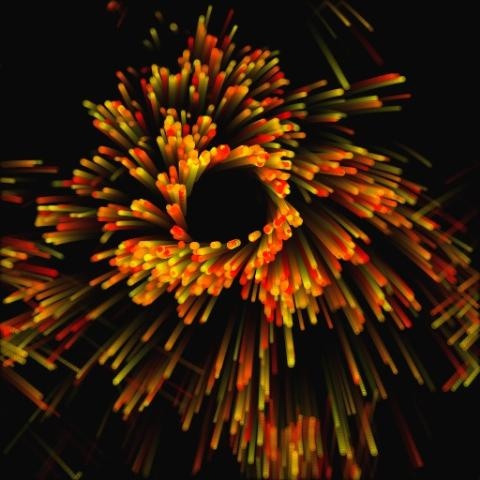## 用JavaScript玩转游戏物理(一)运动学模拟与粒子系统

2010-06-14 16:19 by Milo Yip, ... 阅读, ... 评论, 收藏, 编辑## 运动学模拟

y(t)=\frac{1}{2}gt^2+v_0t+y_0

\begin{align*} \mathbf{v}(t+\Delta t) &= \mathbf{v}(t)+\mathbf{a}(t)\Delta t \\ \mathbf{r}(t+\Delta t) &= \mathbf{r}(t)+\mathbf{v}(t)\Delta t \end{align*}

// Vector2.js
Vector2 = function(x, y) { this.x = x; this.y = y; };

Vector2.prototype = {
copy : function() { return new Vector2(this.x, this.y); },
length : function() { return Math.sqrt(this.x * this.x + this.y * this.y); },
sqrLength : function() { return this.x * this.x + this.y * this.y; },
normalize : function() { var inv = 1/this.length(); return new Vector2(this.x * inv, this.y * inv); },
negate : function() { return new Vector2(-this.x, -this.y); },
add : function(v) { return new Vector2(this.x + v.x, this.y + v.y); },
subtract : function(v) { return new Vector2(this.x - v.x, this.y - v.y); },
multiply : function(f) { return new Vector2(this.x * f, this.y * f); },
divide : function(f) { var invf = 1/f; return new Vector2(this.x * invf, this.y * invf); },
dot : function(v) { return this.x * v.x + this.y * v.y; }
};

Vector2.zero = new Vector2(0, 0);


#### 修改代码试试看

1. 改变起始位置
2. 改变起始速度(包括方向)
3. 改变加速度

## 粒子系统

### 粒子的定义

1. 粒子是独立的，粒子之间互不影响(不碰撞、没有力)
2. 粒子有生命周期，生命结束后会消失
3. 粒子可以理解为空间的一个点，有时候也可以设定半径作为球体和环境碰撞
4. 粒子带有运动状态，也有其他外观状态(例如颜色、影像等)
5. 粒子可以只有线性运动，而不考虑旋转运动(也有例外)

// Particle.js
Particle = function(position, velocity, life, color, size) {
this.position = position;
this.velocity = velocity;
this.acceleration = Vector2.zero;
this.age = 0;
this.life = life;
this.color = color;
this.size = size;
};


### 游戏循环

1. 发射粒子
2. 模拟粒子(粒子老化、碰撞、运动学模拟等等)
3. 渲染粒子

### 生与死

//ParticleSystem.js
function ParticleSystem() {
// Private fields
var that = this;
var particles = new Array();

// Public fields
this.gravity = new Vector2(0, 100);
this.effectors = new Array();

// Public methods

this.emit = function(particle) {
particles.push(particle);
};

// ...
}


function ParticleSystem() {
// ...
this.simulate = function(dt) {
aging(dt);
applyGravity();
applyEffectors();
kinematics(dt);
};

// ...

// Private methods

function aging(dt) {
for (var i = 0; i < particles.length; ) {
var p = particles[i];
p.age += dt;
if (p.age >= p.life)
kill(i);
else
i++;
}
}

function kill(index) {
if (particles.length > 1)
particles[index] = particles[particles.length - 1];
particles.pop();
}
// ...
}


### 运动学模拟

function ParticleSystem() {
// ...
function applyGravity() {
for (var i in particles)
particles[i].acceleration = that.gravity;
}

function kinematics(dt) {
for (var i in particles) {
var p = particles[i];
}
}
// ...
}


### 渲染

function ParticleSystem() {
// ...
this.render = function(ctx) {
for (var i in particles) {
var p = particles[i];
var alpha = 1 - p.age / p.life;
ctx.fillStyle = "rgba("
+ Math.floor(p.color.r * 255) + ","
+ Math.floor(p.color.g * 255) + ","
+ Math.floor(p.color.b * 255) + ","
+ alpha.toFixed(2) + ")";
ctx.beginPath();
ctx.arc(p.position.x, p.position.y, p.size, 0, Math.PI * 2, true);
ctx.closePath();
ctx.fill();
}
}
// ...
}


### 基本粒子系统完成

#### 修改代码试试看

1. 改变发射位置
2. 向上发射，发射范围在90度内
3. 改变生命
4. 改变半径
5. 每帧发射5个粒子

## 简单碰撞

// ChamberBox.js
function ChamberBox(x1, y1, x2, y2) {
this.apply = function(particle) {
if (particle.position.x - particle.size < x1 || particle.position.x + particle.size > x2)
particle.velocity.x = -particle.velocity.x;

if (particle.position.y - particle.size < y1 || particle.position.y + particle.size > y2)
particle.velocity.y = -particle.velocity.y;
};
}